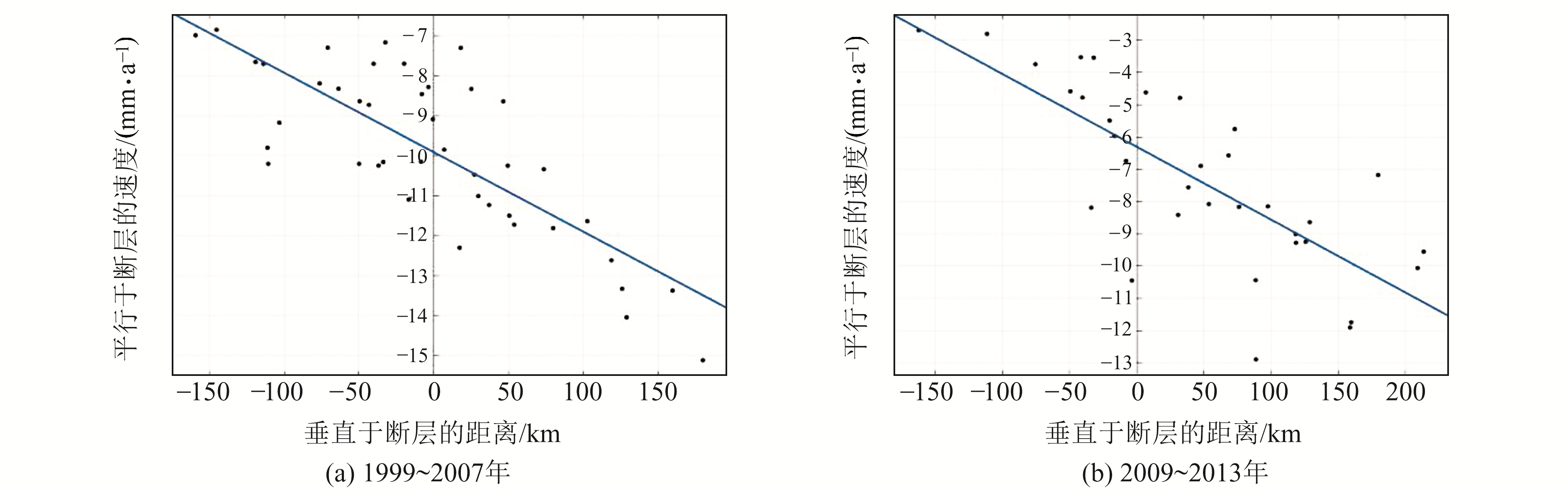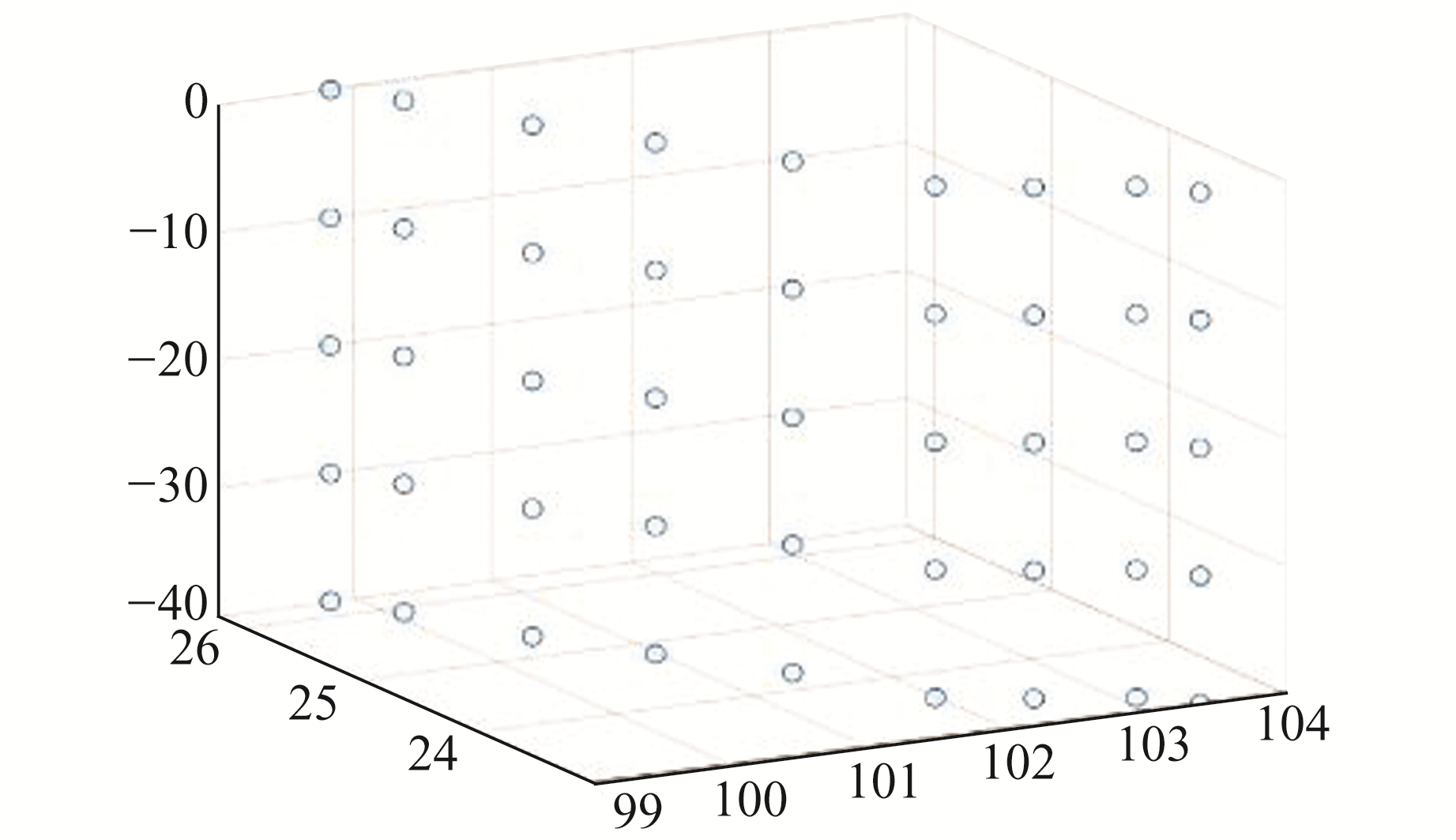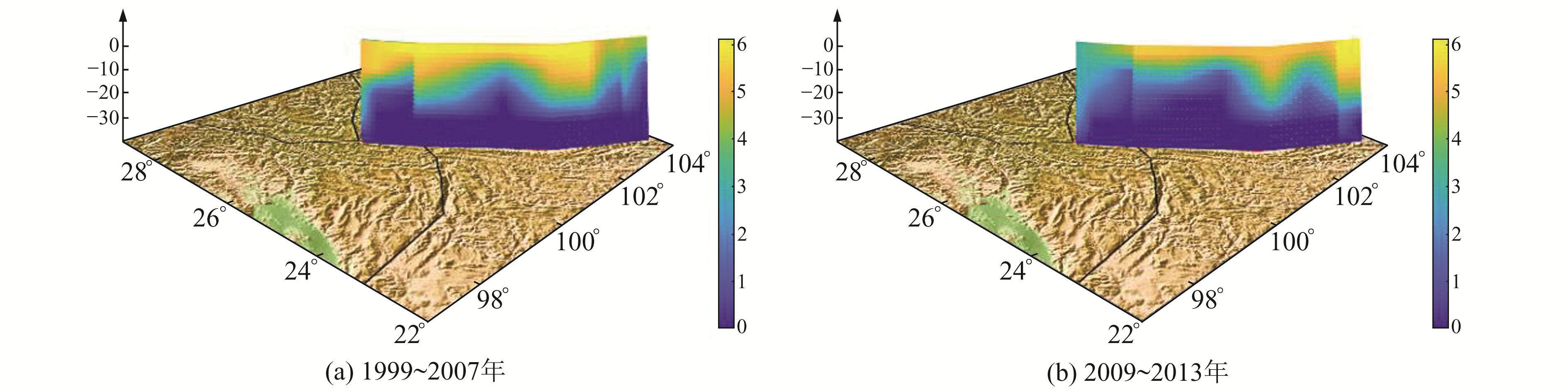﻿ 红河断裂带闭锁程度与滑动亏损分布的GNSS反演文章快速检索 高级检索
 大地测量与地球动力学2021, Vol. 41Issue (3): 296-300  DOI: 10.14075/j.jgg.2021.03.014### 引用本文ZHU Xulin, XU Keke, HOU Zheng, et al. GNSS Inversion of Fault Locking and Fault Slip Deficit of the Red River Fault Zone[J]. Journal of Geodesy and Geodynamics, 2021, 41(3): 296-300.### Foundation support

National Natural Science Foundation of China, No. 41774041.

### About the first author

ZHU Xulin, postgraduate, majors in geodesy and fault zone, E-mail: zhuxvlin@126.com.

### 文章历史

1. 河南理工大学测绘与国土信息工程学院，河南省焦作市世纪大道2001号，454000

1 研究方法

 ${V_{{\rm{sf}}}} = {V_{{\rm{br}}}} + {V_{{\rm{is}}}} + {V_{{\rm{fs}}}}$

 $\begin{array}{l} {V_i}\left( X \right) = \sum\limits_{b = 1}^B {H(X \in {\Delta _b})} \left[ {\mathit{\Omega} _b^R \times X} \right]\cdot i - \\ \sum\limits_{k = 1}^F {\sum\limits_{n = 1}^{{N_k}} {\sum\limits_{j = 1}^2 {{\varphi _{nk}}{G_{ij}}(X, {X_{nk}})\left[ {\mathit{\Omega} _f^h \times {X_{nk}}} \right]\cdot j} } } \end{array}$ (1)

 $\left[ \begin{array}{l} {V_\lambda }\\ {V_\theta } \end{array} \right] = \left[ {\begin{array}{*{20}{c}} {R{\rm{sin}}{\theta _0}\Delta \lambda }&{R\Delta \theta }&0\\ 0&{R{\rm{sin}}{\theta _0}\Delta \lambda }&{R\Delta \theta } \end{array}} \right]\left[ {\begin{array}{*{20}{c}} {\dot \varepsilon _\lambda }\\ {\dot \varepsilon _{\lambda \theta} }\\ {\dot \varepsilon _\theta }\\ \end{array}} \right]$ (2)

 $\chi _n^2 = \frac{{\sum\limits_{i = 1}^n {{{\left( {\frac{{{r_i}}}{{{f_{{\sigma _i}}}}}} \right)}^2}} }}{{{\rm{dof}}}}$ (3)

2 研究区与GNSS观测图 1 研究区速度场 Fig. 1 Velocity field of the study area图 2 GPS速度剖面 Fig. 2 GPS velocity profile
3 实验结果图 3 龙门山断裂带闭锁程度 Fig. 3 Locking degree of Longmenshan fault zone图 4 研究区块体划分 Fig. 4 Blocks in the study area图 5 断层节点模型 Fig. 5 Fault node model图 6 红河断裂带闭锁程度 Fig. 6 Locking degree of Red River fault zone图 7 红河断裂带滑动亏损速率 Fig. 7 Slip deficit velocity of Red River fault zone表 1 红河断裂带滑动速率 Tab. 1 Slip rate of Red River fault zone
4 讨论

5 结语

  汪一鹏, 沈军, 王琪, 等. 川滇块体的侧向挤出问题[J]. 地学前缘, 2003, 10(增): 188-192 (Wang Yipeng, Shen Jun, Wang Qi, et al. On the Lateral Extrusion of Sichuan-Yunnan Block(Chuandian Block)[J]. Earth Science Frontiers, 2003, 10(S): 188-192) (0)  向宏发, 韩竹军, 虢顺民, 等. 红河断裂带大型右旋走滑运动与伴生构造地貌变形[J]. 地震地质, 2004, 26(4): 597-610 (Xiang Hongfa, Han Zhujun, Guo Shunmin, et al. Large-Scale Dextral Strike-Slip Movement and Associated Tectonic Deformation along the Red-River Fault Zone[J]. Seismology and Geology, 2004, 26(4): 597-610) (0)  向宏发, 虢顺民, 张晚霞, 等. 红河断裂带南段中新世以来大型右旋位错量的定量研究[J]. 地震地质, 2007, 29(1): 34-50 (Xiang Hongfa, Guo Shunmin, Zhang Wanxia, et al. Quantitative Study on the Large Scale Dextral Strike-Slip Offset in the Southern Segment of the Red River Fault since Miocene[J]. Seismology and Geology, 2007, 29(1): 34-50) (0)  虢顺民, 向宏发, 计凤桔, 等. 红河断裂带第四纪右旋走滑与尾端拉张转换关系研究[J]. 地震地质, 1996, 18(4): 301-309 (Guo Shunmin, Xiang Hongfa, Ji Fengju, et al. A Study on the Relation between Quaternary Right-Lateral Slip and Tip Extension along the Honghe Fault[J]. Seismology and Geology, 1996, 18(4): 301-309) (0)  李煜航, 郝明, 季灵运, 等. 青藏高原东缘中南部主要活动断裂滑动速率及其地震矩亏损[J]. 地球物理学报, 2014, 57(4): 1 062-1 078 (Li Yuhang, Hao Ming, Ji Lingyun, et al. Fault Slip Rate and Seismic Moment Deficit on Major Active Faults in Mid and South Part of the Eastern Margin of Tibet Plateau[J]. Chinese Journal of Geophysics, 2014, 57(4): 1 062-1 078) (0)  王阎昭, 王恩宁, 沈正康, 等. 基于GPS资料约束反演川滇地区主要断裂现今活动速率[J]. 中国科学: 地球科学, 2008, 38(5): 582-597 (Wang Yanzhao, Wang Enning, Shen Zhengkang, et al. The Inversion of Current Activity Rates of Major Fault in Sichuan-Yunnan Region Based on GPS Data Constraint[J]. Science China: Earth Science, 2008, 38(5): 582-597) (0)  McCaffrey R, Qamar A I, King R W, et al. Fault Locking, Block Rotation and Crustal Deformation in the Pacific Northwest[J]. Geophysical Journal International, 2007, 169(3): 1 315-1 340 DOI:10.1111/j.1365-246X.2007.03371.x (0)  赵静, 江在森, 牛安福, 等. 喜马拉雅主逆冲断层闭锁程度与滑动亏损特征研究[J]. 武汉大学学报: 信息科学版, 2017, 42(12): 1 756-1 764 (Zhao Jing, Jiang Zaisen, Niu Anfu, et al. Characteristics of Fault Locking and Fault Slip Deficit in the Main Himalaya Thrust Fault[J]. Geomatics and Information Science of Wuhan University, 2017, 42(12): 1 756-1 764) (0)  Mao A L, Harrison C G A, Dixon T H. Noise in GPS Coordinate Time Series[J]. Journal of Geophysical Research: Solid Earth, 1999, 104(B2): 2 797-2 816 DOI:10.1029/1998JB900033 (0)  宋淑丽, 朱文耀, 廖新浩. GPS应用于地球动力学研究的进展[J]. 天文学进展, 2003, 21(2): 95-112 (Song Shuli, Zhu Wenyao, Liao Xinhao. Recent Progress of GPS in Geodynamics[J]. Progress in Astronomy, 2003, 21(2): 95-112) (0)  赵静, 江在森, 武艳强, 等. Defnode负位错模型反演结果的可靠性和稳定性分析[J]. 大地测量与地球动力学, 2013, 33(1): 21-24 (Zhao Jing, Jiang Zaisen, Wu Yanqiang, et al. Analysis of Reliability and Stability of Inversion Result with Negative Dislocation Model of Defnode[J]. Journal of Geodesy and Geodynamics, 2013, 33(1): 21-24) (0)  鲁小飞, 谭凯, 赵斌, 等. 汶川地震前后红河断裂闭锁程度和滑动亏损研究[J]. 大地测量与地球动力学, 2019, 39(5): 464-468 (Lu Xiaofei, Tan Kai, Zhao Bin, et al. Research on Fault Locking and Fault Slip Deficit of the Honghe Fault before and after Wenchuan Earthquake[J]. Journal of Geodesy and Geodynamics, 2019, 39(5): 464-468) (0)  刘雷, 桑继镇, 张锡平, 等. 基于GPS和水准资料的西秦岭北缘断裂现今闭锁程度分析[J]. 大地测量与地球动力学, 2019, 39(6): 562-568 (Liu Lei, Sang Jizhen, Zhang Xiping, et al. Analysis of the North Margin of West Qinling Fault Locking Based on GPS and Leveling Data[J]. Journal of Geodesy and Geodynamics, 2019, 39(6): 562-568) (0)  赵静. 块体模型与负位错模型分析川滇地区块体应变与断裂带变形特征[D]. 北京: 中国地震局地震预测研究所, 2012 (Zhao Jing. Analysis of Block Strain and Fault Zone Deformation Characteristics in Sichuan-Yunnan Region Using Block Deformation Model and Negative Dislocation Model[D]. Beijing: Institute of Earthquake Forecasting, CEA, 2012) (0)  闫欢欢, 乔学军, 聂兆生, 等. 利用GPS研究红河断裂带的地壳形变及断层运动特征[J]. 测绘地理信息, 2019, 44(1): 36-40 (Yan Huanhuan, Qiao Xuejun, Nie Zhaosheng, et al. Current Crustal Movement and Fault Dislocation Model of the Red River Fault Zone[J]. Journal of Geomatics, 2019, 44(1): 36-40) (0)
GNSS Inversion of Fault Locking and Fault Slip Deficit of the Red River Fault Zone
ZHU Xulin1XU Keke1     HOU Zheng1     LIU Jipeng1     ZHAO Fuling1     YAO Weizheng1     SHAO Zhenhua1
1. School of Surveying and Land Information Engineering, Henan Polytechnic University, 2001 Shiji Road, Jiaozuo 454000, China
Abstract: In this paper, we use the GPS velocity fields of 1999-2007 and 2009-2013, and the negative dislocation-block model of TDEFNODE to invert fault locking and slip deficit velocity of the Red river fault zone. The results show that the Red river fault zone is dominated by right-lateral strike-slip, with some extension and compression. Before 2008, the average strike-slip rate and dip-slip rate of the Red river fault zone is 3.8±2.5 mm/a and 1.2±2.5 mm/a, the northern and middle segments exhibit tension, and the southern segment exhibits compression. The locking fraction of the northern and middle segments between 0 to 15 km depth are from 0.8 to 0.99, and the slip deficit rate is 4.5 mm/a, for which it is easy to accumulate strain energy quickly. The locking fraction of the southern segment between 0 to 10 km depth is from 0.8 to 0.95, and the slip deficit rate is 4.5 mm/a. After 2008, the fault locking and slip deficit rate in the middle segment decrease, and the fault locking and slip deficit rate in the southern segment increase.
Key words: Red river fault zone; TDEFNODE; fault locking; slip deficit